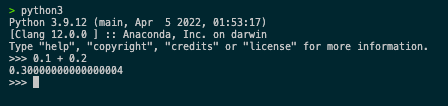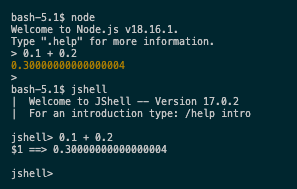It’s easy to forget things that seem the most obvious. For instance, the default behaviour of most programming languages when used for fractional arithmetics lead to inaccurate results due to floating-point precision.

## Example

Consider the following Python code snippet:

``````result = 0.1 + 0.2
``````

The result should be `0.3`, right? Behold:This surprising output occurs due to the way floating-point numbers are represented in binary. It’s not a bug but an inherent limitation in representing some numbers exactly in binary floating-point arithmetic.

Furthermore, this issue is a common phenomenon in some of the other popular languages, including Javascript, Java, etc.## Real-world impact

While this may seem like a trivial issue, it can have a significant impact on the accuracy of computations. For instance, consider a scenario where you are building a financial application that needs to perform precise calculations. In such a case, the imprecise results can lead to incorrect computations and, in turn, incorrect financial decisions.

## Solution

To overcome this issue in Python, we can use the `Decimal` class from the `decimal` module. Here’s an example that provides a precise calculation:

``````from decimal import Decimal

result = Decimal('0.1') + Decimal('0.2')
print(result)  # Output: 0.3
``````

The `Decimal` class provides exact arithmetic and helps to avoid such floating-point issues.

### Pro-tip

In Python at least, `Decimal(0.1)` is not the same as `Decimal('0.1')`. The former is a floating-point number, while the latter is a string. The former will result in the same imprecise output as before, while the latter will provide the correct result. Being aware of this when reading JSON values from an API can save you a lot of time and effort.

## Conclusion

Floating-point precision issues are prevalent in many programming languages. While this can lead to unexpected results, understanding the underlying cause and using appropriate solutions, like the `Decimal` class in Python can provide accurate computations. As we navigate an era increasingly driven by automation and machine learning, in which information and misinformation multiply at an unprecedented rate, the pursuit of accuracy has never been more vital.